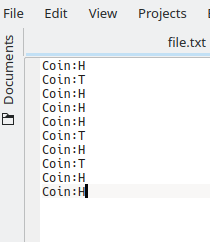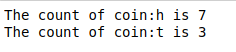Open In App

# Python program to Count the Number of occurrences of a key-value pair in a text file

Given a text file of key-value pairs. The task is to count the number of occurrences of the key-value pairs in the file with Python

## Program to Count the occurrences of a key-value pair

• Naive Approach to Count the Occurrences of a key-value Pair
• Using Python built-in collections.Counter
• Using regular expressions (re module)

Text file:### Naive Approach to Count the Occurrences of a key-value Pair

The approach is very simple. Maintain another dictionary (say d) that will store the count of occurrence of each key-value pair of the file. Store the key-value pair of the text file as a key in the dictionary. Now iterate through the key-value pairs of the file. If the pair is present in the dictionary then increment the value of that pair by one otherwise insert the pair and set its value to one. Below is the implementation.

## Python3

 `# opening text file``f ``=` `open``(``"file.txt"``, ``"r"``)``d ``=` `dict``()` `for` `res ``in` `f:``    ``# removing new line and extra``    ``# space characters``    ``res ``=` `res.strip()` `    ``# changing ase to prevent matching``    ``# errors``    ``res ``=` `res.lower()` `    ``# separating key-value pairs``    ``lines ``=` `res.split()` `    ``for` `line ``in` `lines:` `        ``if` `line ``in` `d:` `            ``# If the key-value pair``            ``# is present in d then``            ``# increment its value by one``            ``d[line] ``=` `d[line]``+``1``        ``else``:` `            ``# Insert the key-value pair``            ``# in the dictionary and sets``            ``# its value to one``            ``d[line] ``=` `1` `f.close()` `# Printing Result``for` `key ``in` `list``(d.keys()):``    ``print``(``"The count of {} is {}"``.``format``(key,d[key]))`

Output :Time complexity: O(n*m), where n is the number of lines in the text file and m is the number of words in each line.
Auxiliary space: O(k), where k is the number of unique key-value pairs in the text file.

### Count Occurrences of a Value in a Python Dictionary using collections.Counter

This method utilizes the `collections.Counter` class, which is a powerful tool for counting occurrences of elements in a collection.

## Python3

 `from` `collections ``import` `Counter` `def` `count_key_value_pairs(file_path, key, value):``    ``with ``open``(file_path, ``'r'``) as ``file``:``        ``lines ``=` `file``.readlines()` `    ``count ``=` `0``    ``for` `line ``in` `lines:``        ``# Assuming the key-value pairs are separated by '=' and there are no spaces around '='``        ``line_key, line_value ``=` `line.strip().split(``'='``)``        ``if` `line_key ``=``=` `key ``and` `line_value ``=``=` `value:``            ``count ``+``=` `1` `    ``return` `count` `if` `__name__ ``=``=` `"__main__"``:``    ``file_path ``=` `"path/to/your/text_file.txt"``    ``key_to_find ``=` `"your_key"``    ``value_to_find ``=` `"your_value"` `    ``occurrences ``=` `count_key_value_pairs(file_path, key_to_find, value_to_find)``    ``print``(f``"Number of occurrences of '{key_to_find}={value_to_find}': {occurrences}"``)`

Output:Time complexity: of O(N * K)
Space complexity: of O(N)

### Count Occurrences of a Value in a Python Dictionary using RegEx

This method utilizes the `re` module to search for key-value pairs in the text file using regular expressions.

## Python3

 `import` `re` `def` `count_key_value_pairs(file_path, key, value):``    ``with ``open``(file_path, ``'r'``) as ``file``:``        ``data ``=` `file``.read()` `    ``pattern ``=` `r``'\b{}={}\b'``.``format``(re.escape(key), re.escape(value))``    ``occurrences ``=` `len``(re.findall(pattern, data))` `    ``return` `occurrences` `if` `__name__ ``=``=` `"__main__"``:``    ``file_path ``=` `"path/to/your/text_file.txt"``    ``key_to_find ``=` `"your_key"``    ``value_to_find ``=` `"your_value"` `    ``occurrences ``=` `count_key_value_pairs(file_path, key_to_find, value_to_find)``    ``print``(f``"Number of occurrences of '{key_to_find}={value_to_find}': {occurrences}"``)`

Output:Time complexity: O(N)
Space complexity: O(N).